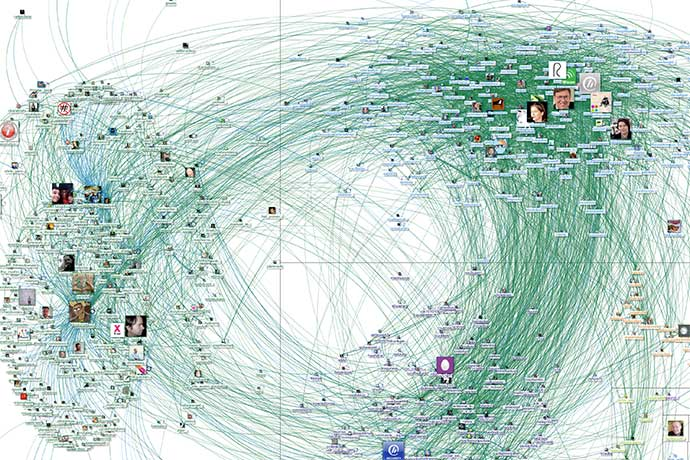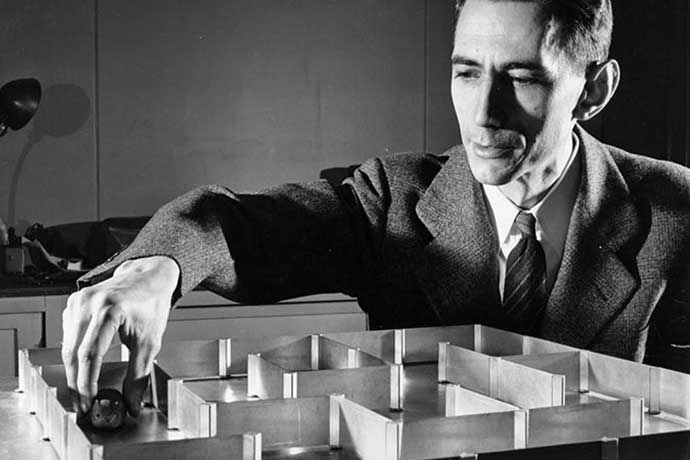#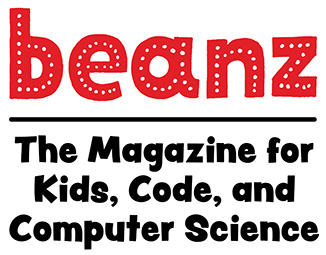beanz Magazine

## 1 and 0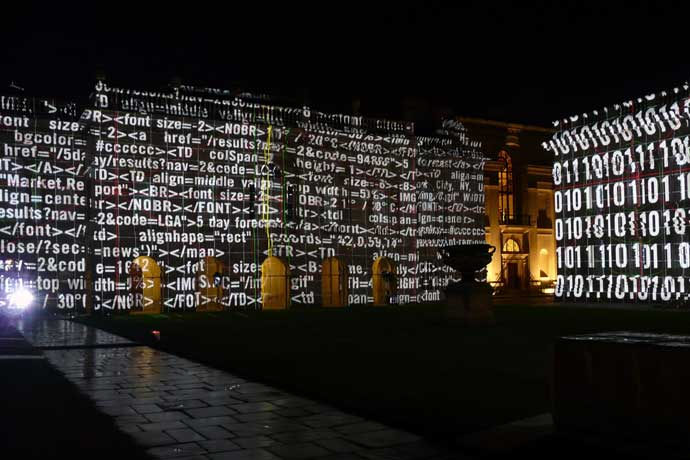David J Morgan on Flickr

Binary numbers, based on 1s and 0s, reflect the practical essence of computer hardware: electricity is either on or off. Learn how to write in binary numbers, and the (not so secret) code to transform English language letters into binary numbers and back again.

When is the letter A not the letter A? Well, computers don't use the letter A. They use the eight character binary number 01000001 to represent A. This binary numbers tutorial describes what binary numbers are and how to calculate them.

Computers transport, calculate, and translate binary numbers because computer hardware circuits only have two electrical states, on or off. These two states can be represented as zero (off) or one (on). All letters of the alphabet, numbers, and symbols are converted to eight character binary numbers as you work with them in software on your computer.

How you create and translate binary numbers is a good way to learn how computers process data at the lowest level, in their hardware circuits.

### The [Not So] Secret Formula

To represent the letter A as 01000001, the computer (and you, to follow along) Â need several basic tools. One tool is an ASCII conversion chart. Without diving into too much technical detail, the ASCII chart maps a unique number between 1 and 255 to all letters of the alphabet capitalized (A-Z) and lower case (a-z), as well as numbers (0-9), spaces, and other special characters. The unique ASCII number that maps to each character, for example, the capital letter A, is used to calculate a unique eight-character binary number, a combination of ones and zeroes like 01000001.

It's basically a two-step secret code. The first step is to grab the unique ASCII number for a letter. The second step is to create a unique eight character binary number, a combination of ones and zeroes to represent the ASCII number.

And, of course, going from the eight character combination of ones and zeros to the letter or character reverses this process: first turn the binary number into a number between 1 and 255 then use the number to look up the letter in the ASCII chart.

### How to Create Binary Numbers

Binary numbers are eight characters in length where every character is either a 1 or 0. The placement of each 1 indicates the value of that position, which is used to calculate the total value of the binary number. Each position of each of the eight characters represents a fixed number value, as shown below.

If you read these Default Value numbers from bottom to top, can you tell how each number immediately above is calculated? They're doubled. So binary numbers start on the bottom with the first position equal to 1. The second position from the bottom has a value 2, the third position 4, and so on.

If you add up all these numbers (1+2+4+8+16+32+64+128), can you guess what number you'll get? 255, the highest number used in the ASCII table. There is a perfect mapping between all possible numbers 1 to 255 in the ASCII table and the calculated values for all possible eight character binary numbers.

To calculate the number value of a binary number, add up the value for each position of all the 1s in the eight character number. The number 01000001, for example, is converted to 64 + 1 or 65. The ones in this binary number are in the first and seventh positions, counting from the bottom to top, or reading right to left. The first position has an assigned value of 1 while the seventh position has an assigned value of 64.

### Let's Convert a Letter to a Binary Number

Now that you know the [not so] secret formula to convert letters to unique ASCII numbers to binary numbers, and how to create binary numbers, let's do the whole process step by step. Let's start with the letter C.

First, we need to use an ASCII chart like this one below to look up the unique number assigned to the uppercase letter C. The unique decimal number to use is 67.

Decimal Character Decimal Character Decimal Character
32 Space 64 @ 96 `
33 ! 65 A 97 a
34 66 B 98 b
35 # 67 C 99 c
36 \$ 68 D 100 d
37 % 69 E 101 e
38 & 70 F 102 f
39 71 G 103 g
40 ( 72 H 104 h
41 ) 73 I 105 i
42 * 74 J 106 j
43 + 75 K 107 k
44 , 76 L 108 l
45 77 M 109 m
46 . 78 N 110 n
47 / 79 O 111 o
48 0 80 P 112 p
49 1 81 Q 113 q
50 2 82 R 114 r
51 3 83 S 115 s
52 4 84 T 116 t
53 5 85 U 117 u
54 6 86 V 118 v
55 7 87 W 119 w
56 8 88 X 120 x
57 9 89 Y 121 y
58 : 90 Z 122 z
59 ; 91 [ 123 {
60 < 92 124 |
61 = 93 ] 125 }
62 > 94 ^ 126 ~
63 ? 95 _ 127 DEL

To convert the number for C, 67, into a binary number:

Remember how binary numbers are read bottom to top, first position and Default Value to top position and Default Value, with each of the eight character positions assigned a unique number value? With the chart below, what combination of values would equal 67?

You're correct if you said the Default Values 1 plus 2 plus 64 would equal 67, the ASCII number for the capital letter C. So let's change the first, second, and seventh position zeroes to ones, counted from right to left. The binary number is for the capital letter C is:

Can you decode this binary number? Add up the 1s to get 64+16+4 or 84. Look up the decimal number 84 in the ASCII chart to find the letter represented below:

If you converted this binary number to the capital letter T, you're correct. Here is the letter A as a binary number to represent the ASCII decimal number for A, which is 65:

If we combine the binary numbers we've looked at so far, we can spell CAT:

01000011 01000001 01010100

### Bonus: Pseudo-Code to Design a Binary Number Converter

With an understanding of how letters and numbers are converted to binary numbers, and back, let's look at how we might create a software application to make these conversions on the fly. The application has no real value. But it provides a chance to discuss how a process can be converted to software.

Instead of actual code, however, we'll write up a series of statements or pseudo-code.

Let's take the word cat to start. What process do we need to convert these letters automatically into binary numbers? Here's one possible set of steps we could code:

1. Break the word into individual letters.
2. For each letter, look up the ASCII number value mapped to the letter.
3. For each ASCII number value, convert to a binary number.
4. For each binary number, save the binary number value. If it is the first binary number, create the initial binary number value; if a binary number value exists, add the new binary number to the end of the value.

Imagine if we skipped the last step: what would be the result of these steps? We'd only have the last binary number, for the lower case letter t in cat. It's important we capture each binary number as they are created.

Other observations about this pseudo-code process? We need to distinguish between capital letters and lower case letters, don't we? Otherwise, our binary number conversion might translate from binary number to ASCII letters as CAT or cAT or Cat. Our lookup to match letters to the ASCII table might grab the wrong number.

### Bonus Bonus: A Final Puzzle

Can you decode the phrase in this set of binary numbers? Remember, these are eight character blocks of 1s and 0s.

01000011 01101111 01100100 01100101   01101001 01110011   01010000 01101111 01100101 01110100 01110010 01111001

Here’s a fairly easy way to convert any letter into a binary number. Grab a calculator, find the ASCII decimal value for the letter, from the chart above, then look at the binary number chart for the nearest value to the decimal value. Subtract the nearest number Default Value in the binary chart to get a remainder value. Look for the nearest binary Default Value for the remainder. Repeat until you run out of binary values.

If you’re clever, you’ll also note the sum of the values below any of the eight Default Values equals one less than the value: so below the binary value 4 are the values 2 and 1 which equal 3. Below the binary value of 8 are 4, 2, and 1 which equal 7. This also can help convert letters to binary numbers. If your remainder is 7, for example, then you know to put a 1 at the 4, 2, and 1 positions to create that part of your binary number.

To convert binary numbers to letters, just grab a piece of paper and a pen or pencil and add up the binary values of all the 1s. Then look up your total number as an ASCII decimal in the chart above.

Here’s a hint to help determine if you have solved the binary numbers above correctly: I majored in American poetry in college and love the old tagline used for WordPress publishing software.

#### Binary Number Worksheet (Excel format)

https://www.kidscodecs.com/binary-numbers-converter

#### Binary Numbers Worksheet (PDF)

https://www.kidscodecs.com/binary-numbers-worksheet

#### Binary Converter

http://www.rapidtables.com/convert/number/binary-converter.htm

#### Binary Numbers (Wikipedia)

https://en.wikipedia.org/wiki/Binary_number

#### ASCII Table

http://www.asciitable.com/

(Visited 843 times, 22 visits today)

### Also In The September 2013 Issue### 1 and 0

Binary numbers, based on 1s and 0s, reflect the practical essence of computer hardware: electricity is either on or off. Learn how to write in binary numbers, and the (not so secret) code to transform English language letters into binary numbers and back again.### Simon Haughton Talks about Kids, Python, and Computer Science

Simon recently wrote a short ebook, A children's guide to Python programming, to teach kids ages 5-8 computer programming with Python..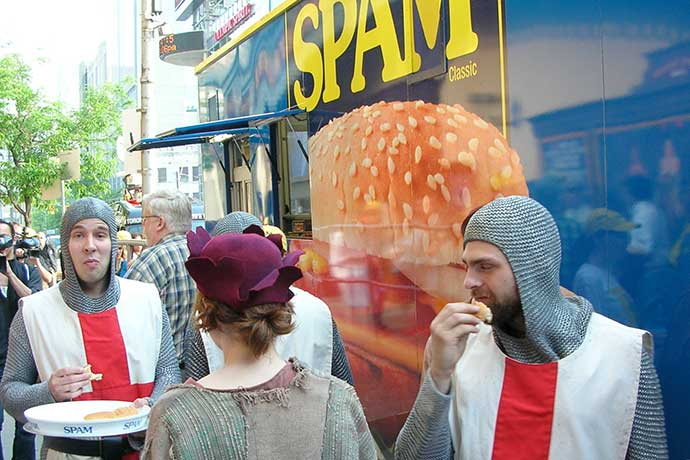### Python

Named after Monty Python, this language is designed to be simple yet powerful, easy to code with lots of features.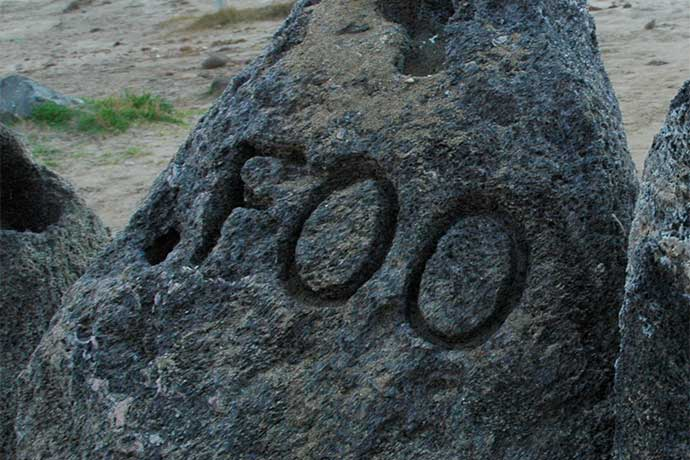### Variables

In most or all software programming languages, variables work like containers to hold numbers, phrases, or other important stuff used in several places in your code. Here's how they work in several common languages.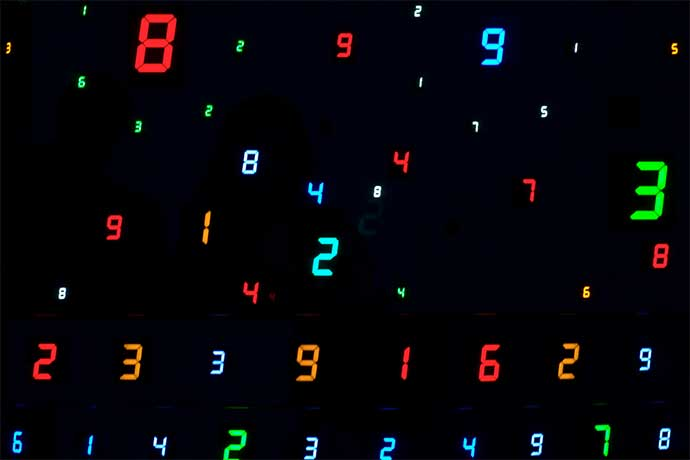### Happy Numbers

Some numbers simply have a positive attitude. They're fun to play with.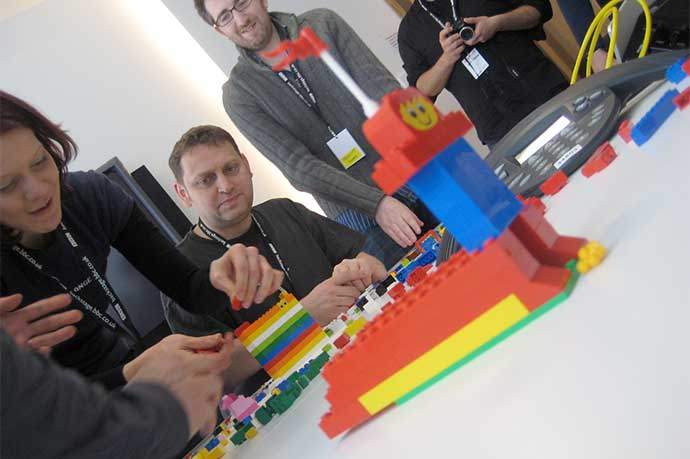### Local Coding and Technology Groups

Girls Who Code, CoderDojo, and other local groups are great places to learn how to program, meet people, and help others learn.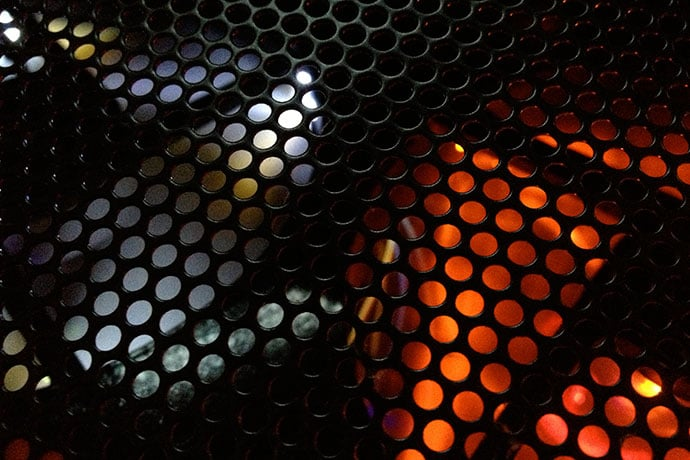### How to Build a Computer

Building your own computer is a great way to not only save money, and get more processing power, but also to learn about the less obvious parts of software programming.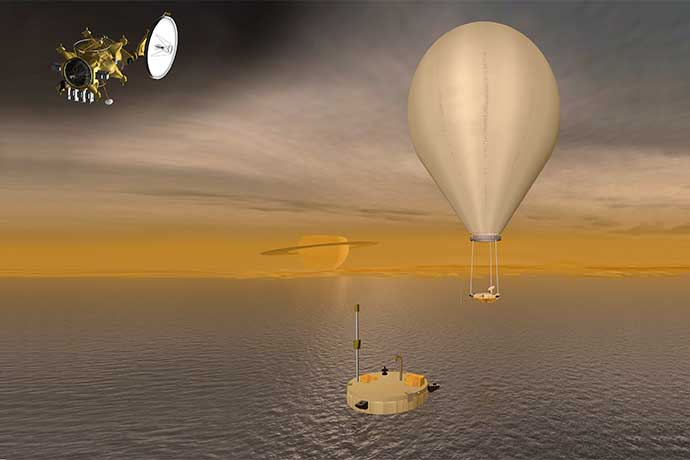### News Wire Stories for September 2013

Interesting stories about computer science, software programming, and technology for the month of August 2013.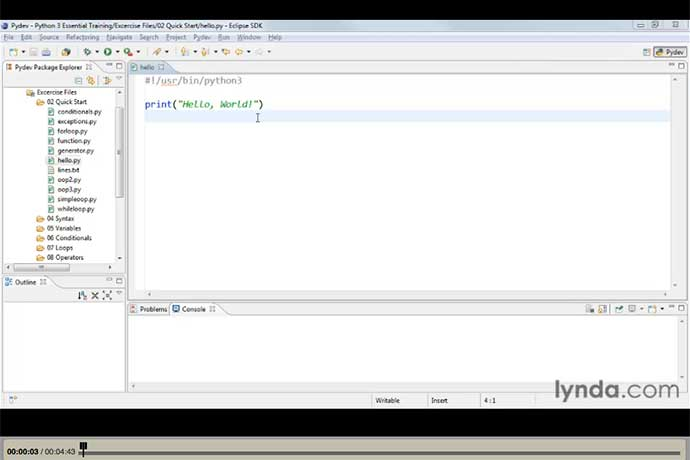### Online Coding Schools

There are plenty of places online to learn one or more software programming languages. Here's a short list with some guidelines to evaluate all your options.

### Computer science is no more about computers than astronomy is about telescopes, biology about microscopes, or chemistry about beakers and test tubes. Science is not about tools. It is about how we use them, and what we find out when we do.